Practicing Success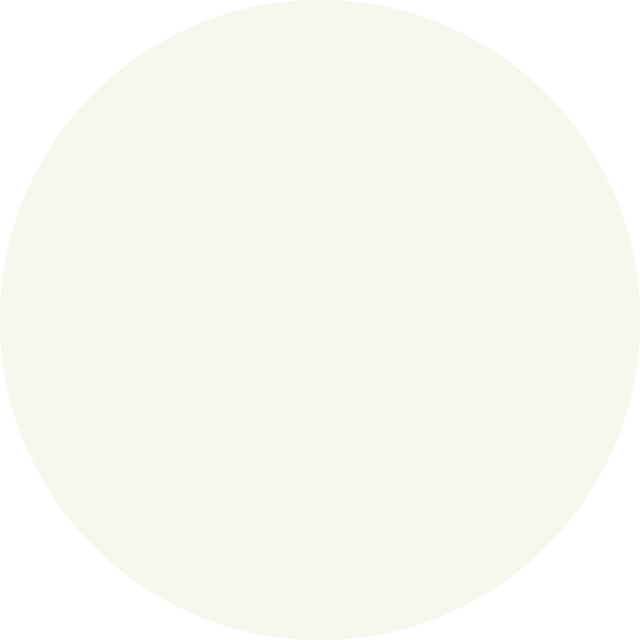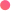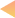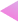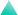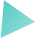COMPLETE SYLLABUS FOR

### CUET UG :: Physics

♦   Mock Papers

### Complete Syllabus

Physics syllabus for CUET comprises 10 units and their respective sub-units. All the topics are interconnected and equally important. The detailed syllabus is given in the table below:

SL. No. UNIT SUB-UNIT

Unit.1.

Electrostatics

Electric charges and their conservation. Coulomb’s law – force between two point charges, forces between

multiple charges; superposition principle, and continuous charge distribution.

Electric field, electric field due to a point charge, electric field lines; electric dipole, electric field due to a

dipole; torque on a dipole in a uniform electric field.

Electric flux, a statement of Gauss’s theorem and its applications to finding field due to infinitely long straight wire, uniformly charged infinite plane sheet, and uniformly charged thin spherical shell (field inside and

outside).

Electric potential, potential difference, electric potential due to a point charge, a dipole and system of charges; equipotential surfaces, the electrical potential energy of a system of two point charges, and electric dipoles in an electrostatic field.

Conductors and insulators, free charges, and bound charges inside a conductor. Dielectrics and electric polarisation, capacitors and capacitance, the combination of capacitors in series and in parallel, the capacitance of a parallel plate capacitor with and without dielectric medium between the plates, energy

stored in a capacitor, Van de Graaff generator.

Unit.2.

Current Electricity

Electric current, the flow of electric charges in a metallic conductor, drift velocity and mobility, and their relation with electric current; Ohm’s law, electrical resistance, V-I characteristics (linear and nonlinear), electrical energy and power, electrical resistivity and conductivity.

Carbon resistors, colour code for carbon resistors; series and parallel combinations of resistors; temperature dependence of resistance.

The internal resistance of a cell, potential difference, and emf of a cell, combination of cells in series and in parallel.

Kirchhoff ’s laws and simple applications. Wheatstone bridge, metre bridge.

Potentiometer – principle, and applications to measure potential difference, and for comparing emf of two cells; measurement of internal resistance of a cell.

Unit.3.

Magnetic Effects of Current and Magnetism

Concept of the magnetic field, Oersted’s experiment. Biot - Savart law and its application to the current-carrying circular loop.

Ampere’s law and its applications to infinitely long straight wire, straight and toroidal solenoids. Force on a moving charge in uniform magnetic and electric fields. Cyclotron.

Force on a current-carrying conductor in a uniform magnetic field. The force between two parallel current-carrying conductors – definition of ampere. Torque experienced by a current loop in a magnetic field; moving coil galvanometer – its current sensitivity and conversion to ammeter and voltmeter.

Current loop as a magnetic dipole and its magnetic dipole moment. The magnetic dipole moment of a revolving electron. Magnetic field intensity is due to a magnetic dipole (bar magnet) along its axis and perpendicular to its axis.

Torque on a magnetic dipole (bar magnet) in a uniform magnetic field; bar magnet as an equivalent solenoid, magnetic field lines; Earth’s magnetic field and magnetic elements.

Para-, dia- and ferromagnetic substances, with examples. Electromagnets and factors affecting their strengths. Permanent magnets.

Unit.4.

Electromagnetic Induction and Alternating Currents

Electromagnetic induction; Faraday’s law, induced emf and current; Lenz’s Law, Eddy currents. Self and mutual inductance.

Alternating currents, peak and RMS value of alternating current/voltage; reactance and impedance; LC oscillations (qualitative treatment only), LCR series circuit, resonance; power in AC circuits, wattles current.

AC generator and transformer.

Unit.5.

Electromagnetic Waves

Need for displacement current. Electromagnetic waves and their characteristics (qualitative ideas only).

Transverse nature of electromagnetic waves.

Electromagnetic spectrum (radio waves, microwaves, infrared, visible, ultraviolet, x-rays, gamma rays) includes elementary facts about their uses.

Unit.6.

Optics

Reflection of light, spherical mirrors, mirror formula. Refraction of light, total internal reflection, and its applications, optical fibres, refraction at spherical surfaces, lenses, thin lens formula, lens maker's formula. Magnification, power of a lens, combination of thin lenses in contact combination of a lens and a mirror. Refraction and dispersion of light through a prism.

Scattering of light–blue colour of the sky and reddish appearance of the sun at sunrise and sunset.

Optical instruments: Human eye, image formation, and accommodation, correction of eye defects (myopia and hypermetropia) using lenses.

Microscopes and astronomical telescopes (reflecting and refracting) and their magnifying powers.

Wave optics: Wavefront and Huygens’ principle, reflection, and refraction of plane waves at a plane surface using wavefronts.

Proof of laws of reflection and refraction using Huygens’ principle.

Interference, Young’s double hole experiment and expression for fringe width, coherent sources, and sustained interference of light.

Diffraction due to a single slit, width of central maximum.

Resolving the power of microscopes and astronomical telescopes. Polarisation, plane polarised light; Brewster’s law, uses of plane polarised light and Polaroids.

Unit.7.

Dual Nature of Matter and Radiation

Photoelectric effect, Hertz and Lenard’s observations;  Einstein’s photoelectric equation – particle nature of light.

Matter wave-wave nature of particles, de Broglie relation. Davisson-Germer experiment

(experimental details should be omitted; only the conclusion should be explained.)

Unit.8

Atoms and Nuclei

Alpha - particle scattering experiment; Rutherford’s model of atom; Bohr model, energy levels, hydrogen spectrum. Composition and size of nucleus, atomic masses, isotopes, isobars; isotones.

Radioactivity – alpha, beta, and gamma particles/rays, and their properties; radioactive decay law. Mass energy relation, mass defect; binding energy per nucleon and its variation with mass number; nuclear fission

and fusion.

Unit.9.

Electronic Devices

Energy bands in solids (qualitative ideas only), conductors, insulators, and semiconductors;

semiconductor diode – I-V characteristics in forward and reverse bias, diode as a rectifier; I-V characteristics of LED, photodiode, solar cell, and Zener diode; Zener diode as a voltage regulator. Junction transistor, transistor action, characteristics of a transistor; transistor as an amplifier (common emitter configuration)

and oscillator. Logic gates (OR, AND, NOT, NAND and NOR). Transistor as a switch.

Unit.10.

Communication Systems

Elements of a communication system (block diagram only); bandwidth of signals (speech, TV, and digital data); bandwidth of transmission medium. Propagation of electromagnetic waves in the atmosphere, sky, and space wave propagation. Need for modulation. Production and detection of an

amplitude-modulated wave.